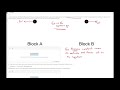# Search Engine

AP Physics 1 Exam Free Response Example.# Video Informations

 Title : AP Physics 1 Exam Free Response Example. Duration : 8m 45s Uploader : Math And Physics Tutor Added On: : 20 April, 2020 Views : 13293 times Likes : 274 Dislikes: : 6 Source : YouTube

# Descriptions

Two identical blocks, A and B , are on a horizontal surface, as shown above. There is negligible friction between the surface and the blocks. As shown in Figure 1, block A is initially moving toward block B with speed vA=v0 and block B is initially at rest. Figure 2 shows the blocks in contact as they collide a short time later. In both figures, the location of the center of mass of the two-block system is indicated by the X that is labeled CM. (a) i. On each of the dots, which represent the blocks while they are in contact during the collision, draw a single arrow to indicate the direction of the net force, if any, exerted on each block. The net force must be represented by a distinct arrow starting on, and pointing away from, the appropriate dot. If the net force is zero for either block, write “zero” under the dot representing that block. ii. During the time that the blocks are in contact, describe whether the center of mass of the two-block system is speeding up, slowing down, or staying the same. ____ Speeding up ____ Slowing down ____ Staying the same Briefly describe, in terms of a basic law of physics, whether the center of mass of the two-block system is speeding up, slowing down, or staying the same. (b) The surface is tilted to an angle of 37 degrees from the horizontal, as shown above in Figure 3. The blocks are each given a push so that at the instant shown, they are moving toward each other. i. Each block has a mass of 0.20kg. Calculate the magnitude of the net force exerted on the two-block system at the instant shown above. ii. At the instant shown in Figure 3, the blocks are moving toward each other with the same speed of 0.35m/s. The blocks collide 0.50 seconds later. What is the speed of the two-block system’s center of mass just before the blocks collide? If there is a topic you want me to do leave them in the comments below. #physicstutor #mathtutor